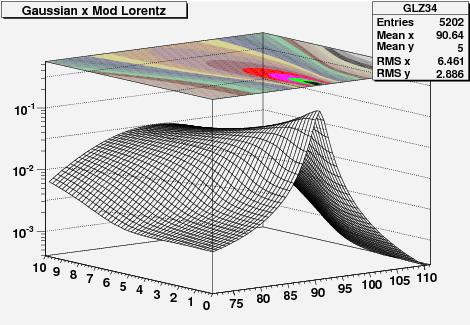## More on the Z lineshape at LHCDecember 19, 2008

Posted by dorigo in personal, physics, science.
Tags: , , ,

Yesterday I posted a nice-looking graph without abounding in explanations on how I determined it. Let me fill that gap here today.

A short introduction

Z bosons will be produced copiously at the LHC in proton-proton collisions. What happens is that a quark from one proton hits an antiquark of the same flavour in the other proton, and the pair annihilates, producing the Z. This is a weak interaction: a relatively rare process, because weak interactions are much less frequent than strong interactions. Quarks carry colour charge as well as weak hypercharge, and most of the times when they hit each other what “reacts” is their colour, not their hypercharge. Similarly, when you meet  John at the coffee machine you discuss football more often than chinese checkers: in particle physics terms, that is because your football coupling with John is stronger than your chinese-checkers coupling.

## Result now, explanation laterDecember 18, 2008

Posted by dorigo in personal, physics, science.
Tags: , , ,

Tonight I feel accomplished, since I have completed a crucial update of the cornerstone of the algorithm which provides the calibration of the CMS momentum scale. I have no time to discuss the details tonight, but I will share with you the final result of a complicated multi-part calculation (at least, for my mediocre standards): the probability distribution function of measuring the Z boson mass at a certain value$M$, using the quadrimomenta of two muon tracks which correspond to an estimated mass resolution$\sigma_M$, when the rapidity of the Z boson is$Y_Z$.

The above might -and should, if you are not a HEP physicist- sound rather meaningless, but the family of two-dimensional functions$P(M,\sigma_M)_Y$ is needed for a precise calibration of the CMS tracker. They can be derived by convoluting the production cross-section of Z bosons$\sigma_M$ at a given rapidity$Y$ with the proton’s parton distribution functions using a factorization integral, and then convoluting the resulting functions with a smearing Gaussian distribution of width$\sigma_M$.

Still confused ? No worry. Today I will only show one sample result – the probability distribution as a function of$M$ and$\sigma_M$ for Z bosons produced at a rapidity$2.8< |Y| <2.9$, and tomorrow I will explain in simple terms how I obtained that curve and the other 39 I have extracted today.In the three-dimensional graph above, one axis has the reconstructed mass of muon pairs$M$ (from 71 to 111 GeV), the other has the expected mass resolution$\sigma_M$ (from 0 to 10 GeV). The height of the function is the probability of observing the mass value$M$, if the expected resolution is$\sigma_M$. On top of the graph one also sees in colors the curves of equal probability displayed on a projected plane. It will not escape to the keen eye that the function is asymmetric in mass around its peak: that is entirely the effect of the parton distribution functions…

## Hectic weekDecember 4, 2008

Posted by dorigo in personal, physics, science.
Tags: , , , ,
The second is the search for Higgs boson decays in the final state arising when H decays to two Z bosons, and one of the Z decays to a lepton pair, while the other decays to a pair of jets. Usually this final state, which is very hard to exploit at low Higgs masses due to the large backgrounds, is used for high-mass searches only (above 200 GeV). We want to extend it to lower masses, where the Higgs is more likely to be, using the$Z \to b \bar b$ decay, which Mia and I have a lot of experience in detecting in hadronic environments. Mia will present some results of this study tomorrow at CERN, so we have been working at this heavily this week.